Test: Thermodynamics And Thermochemistry - 3

# Test: Thermodynamics And Thermochemistry - 3

Test Description

## 30 Questions MCQ Test Physical Chemistry | Test: Thermodynamics And Thermochemistry - 3

Test: Thermodynamics And Thermochemistry - 3 for Chemistry 2023 is part of Physical Chemistry preparation. The Test: Thermodynamics And Thermochemistry - 3 questions and answers have been prepared according to the Chemistry exam syllabus.The Test: Thermodynamics And Thermochemistry - 3 MCQs are made for Chemistry 2023 Exam. Find important definitions, questions, notes, meanings, examples, exercises, MCQs and online tests for Test: Thermodynamics And Thermochemistry - 3 below.
Solutions of Test: Thermodynamics And Thermochemistry - 3 questions in English are available as part of our Physical Chemistry for Chemistry & Test: Thermodynamics And Thermochemistry - 3 solutions in Hindi for Physical Chemistry course. Download more important topics, notes, lectures and mock test series for Chemistry Exam by signing up for free. Attempt Test: Thermodynamics And Thermochemistry - 3 | 30 questions in 90 minutes | Mock test for Chemistry preparation | Free important questions MCQ to study Physical Chemistry for Chemistry Exam | Download free PDF with solutions
 1 Crore+ students have signed up on EduRev. Have you?
Test: Thermodynamics And Thermochemistry - 3 - Question 1

### Match the following: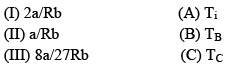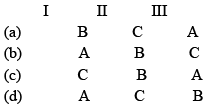Test: Thermodynamics And Thermochemistry - 3 - Question 2

### dU for Isothermal Process for Vander Waals Gas and Ideal Gas respectively are:

Detailed Solution for Test: Thermodynamics And Thermochemistry - 3 - Question 2

Explanation : a) U = (v,T)

dU = (du/dv)T dv + (dU/dT) dT

here, (dU/dT)v = nCv    and    (dU/dV)T = an2/v2

for isothermal process dT = 0

dU = an2/v2 dv

Integrating it,

∫(U2 to U1)dU = ∫(V2 to V1)an2/v2 dv

(U2 - U1) = an2[-1/v](V2 to V1)

ΔU = -an2[1/v2 - 1/v1]

b)we can express the relationship between internal energy and temperature as:

ΔU = CvdT

internal energy is a function of temperature because internal energy of ideal gas comprises of molecular kinetic energy which further depends on the temperature and hence,

For isothermal process, dT = 0, then ΔU = 0

Test: Thermodynamics And Thermochemistry - 3 - Question 3

### For an irreversible isothermal expansion of a perfect gas from Vi to Vt, the change in entropy of the gas is:

Test: Thermodynamics And Thermochemistry - 3 - Question 4

Match the following: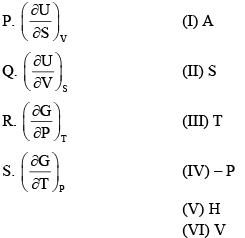Test: Thermodynamics And Thermochemistry - 3 - Question 5

Standard entropy of crystalline carbon monoxide (in J/mol) at 00K is around:

Test: Thermodynamics And Thermochemistry - 3 - Question 6

If a gas obeys the equation of state P(V-nb)= nRT, the ratio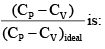Test: Thermodynamics And Thermochemistry - 3 - Question 7

Two moles of a monoatomic perfect gas initially 4.0 bars and 470C undergoes reversible expansion in an insulated container. The temperature at which the pressure reduces to 3.0 bar is:

Test: Thermodynamics And Thermochemistry - 3 - Question 8

Choose the correct criterion of spontaneity in terms of the properties of the system alone:

Test: Thermodynamics And Thermochemistry - 3 - Question 9

For the reaction: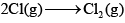The thermodynamic properties:

Test: Thermodynamics And Thermochemistry - 3 - Question 10

The change in entropy when one mole of an ideal gas is compressed to one-fourth of its initial volume and simultaneously heated to twice its initial temperature is:

Test: Thermodynamics And Thermochemistry - 3 - Question 11

The internal pressure,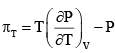for one mole of a Vander Waals gas is:

Test: Thermodynamics And Thermochemistry - 3 - Question 12

Consider the following P–V diagram for an ideal gas that follows the diagonal path from A to B: The work done (in atm–L) on the gas in the process is: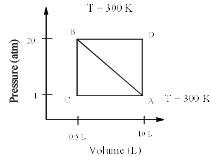Test: Thermodynamics And Thermochemistry - 3 - Question 13

One mole of an ideal gas (CP = 29.234 JK–1mol–1) is expanded reversibly and adiabatically from 1dm3 to 10 dm3. The initial temperature is 750 K, the final temperature will be:

Test: Thermodynamics And Thermochemistry - 3 - Question 14

Using Joule–Thompson effect, find the current expression for Δ T:

Test: Thermodynamics And Thermochemistry - 3 - Question 15

The relationship between volume change in an isothermal process (Δ Vi) and an adiabatic process (Δ Va) for a pressure change from P1 to P2 is:

Test: Thermodynamics And Thermochemistry - 3 - Question 16

An adiabat ic process is an:

Test: Thermodynamics And Thermochemistry - 3 - Question 17

For one mole of an ideal gas,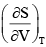is equal to:

Test: Thermodynamics And Thermochemistry - 3 - Question 18

Which one of the following relations is correct:

Test: Thermodynamics And Thermochemistry - 3 - Question 19

The change in enthalpy with respect to change in pressure at constant temperature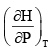is :

Test: Thermodynamics And Thermochemistry - 3 - Question 20

Find the value of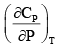for ideal gas:

Test: Thermodynamics And Thermochemistry - 3 - Question 21

The change in entropy when three moles of argon gas are heated at constant volume from 200 K to 300 K is:

Test: Thermodynamics And Thermochemistry - 3 - Question 22

For a system of constant composition, the pressure P is given by:

Detailed Solution for Test: Thermodynamics And Thermochemistry - 3 - Question 22

du = tds - pdv            at constant entropy ds = 0

so du = -pdv

du/dv=-p

Test: Thermodynamics And Thermochemistry - 3 - Question 23

Calculate the coefficient of performance (β) of an engine operating between 1100C and 250C. (The efficiency of Carnot Engine is 22.2%):

Test: Thermodynamics And Thermochemistry - 3 - Question 24

Δ H and Δ U for the reaction: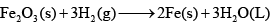At constant temperature are related as:

Test: Thermodynamics And Thermochemistry - 3 - Question 25

Consider the following statements:

(I) The work done in the reversible isothermal expansion of an ideal gas is greater than that for Vander Waals Gas
(II) When an ideal gas undergoes adiabatic expansion, internal energy increases Which of the statement is true?

Test: Thermodynamics And Thermochemistry - 3 - Question 26

Match the List-I with List-II and select the correct answers: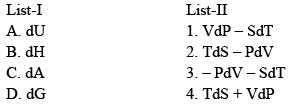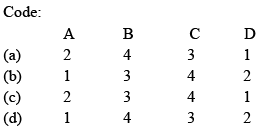Test: Thermodynamics And Thermochemistry - 3 - Question 27

Which of the following statement is false:

Test: Thermodynamics And Thermochemistry - 3 - Question 28

One mole of an ideal gas at 300 K is expanded isothermally from an initial volume of 1 L to 10 L. The Δ U for the process is: R = 2 cal/k–mole

Test: Thermodynamics And Thermochemistry - 3 - Question 29

When 1 mole of a gas is heated at constant volume temperature is raised from 298 K to 308 K. Heat supplied to the gas is 500 J. Then, which statement is correct:

Test: Thermodynamics And Thermochemistry - 3 - Question 30

Two moles of an ideal gas are expanded isothermally and reversibly from 1 L to 10 L at 300 KJ. The enthalpy change (in KJ) for the process is:

## Physical Chemistry

85 videos|131 docs|67 tests
 Use Code STAYHOME200 and get INR 200 additional OFF Use Coupon Code
Information about Test: Thermodynamics And Thermochemistry - 3 Page
In this test you can find the Exam questions for Test: Thermodynamics And Thermochemistry - 3 solved & explained in the simplest way possible. Besides giving Questions and answers for Test: Thermodynamics And Thermochemistry - 3, EduRev gives you an ample number of Online tests for practice

## Physical Chemistry

85 videos|131 docs|67 tests